Miscellaneous

Chapter 4 Class 12 Determinants
Serial order wise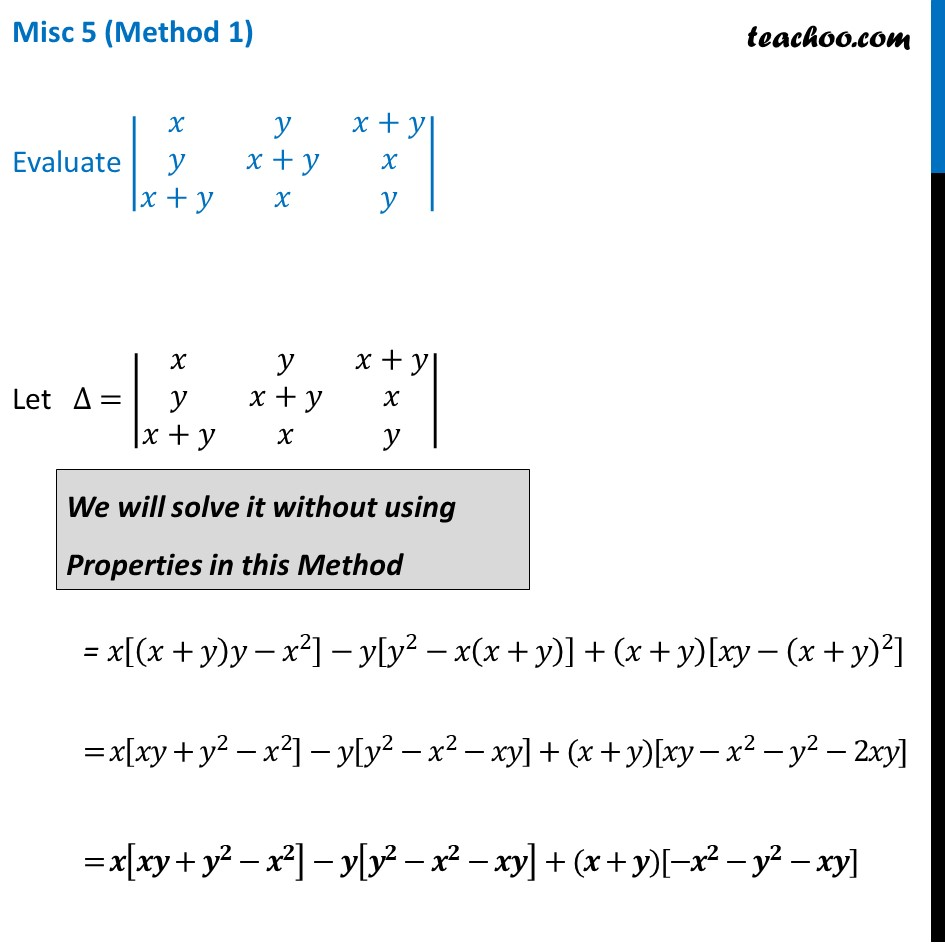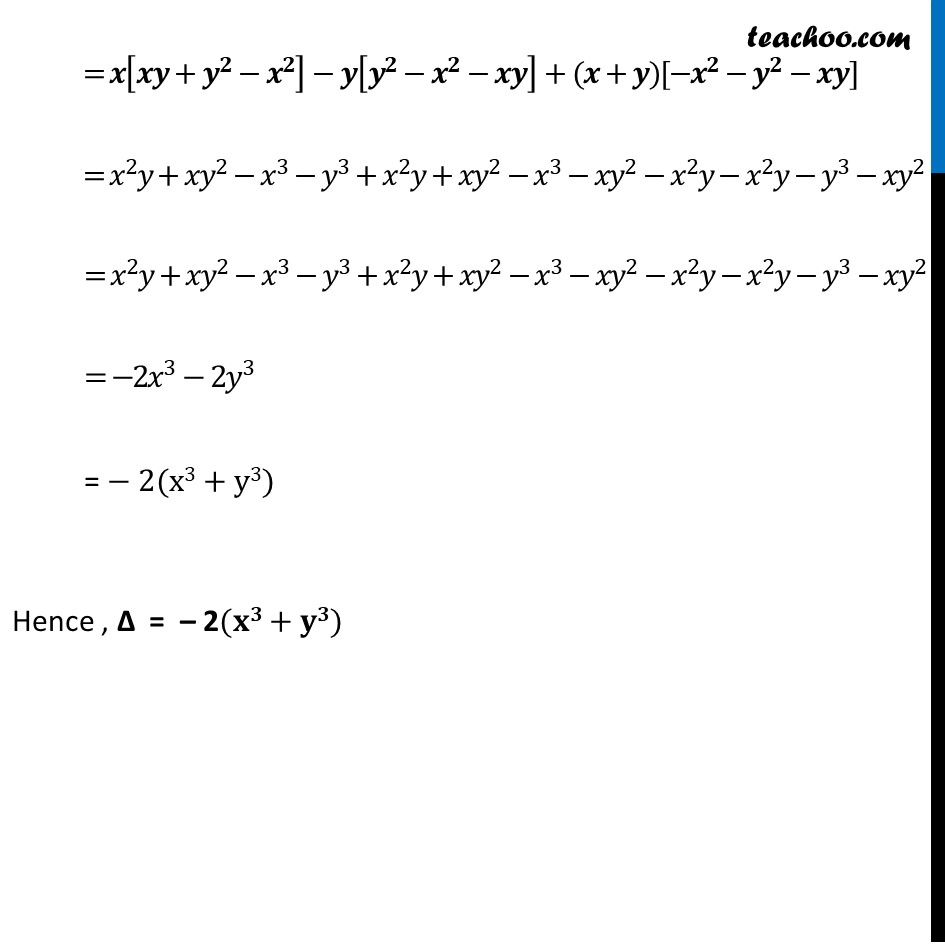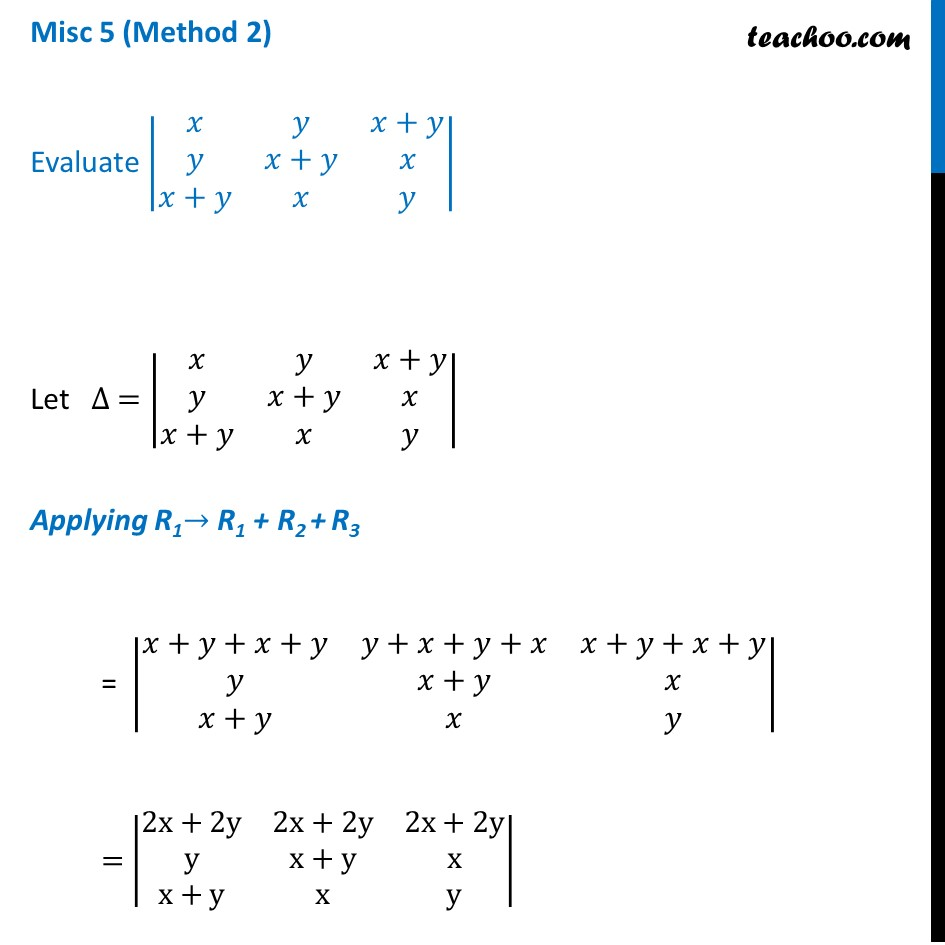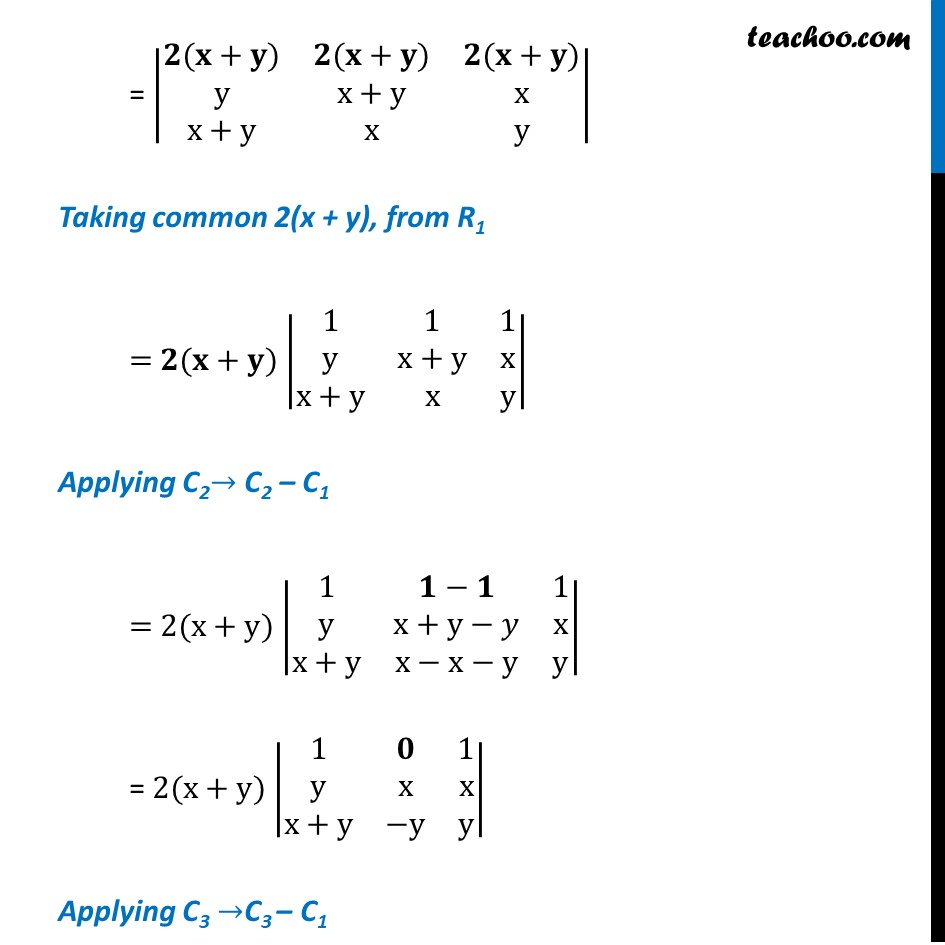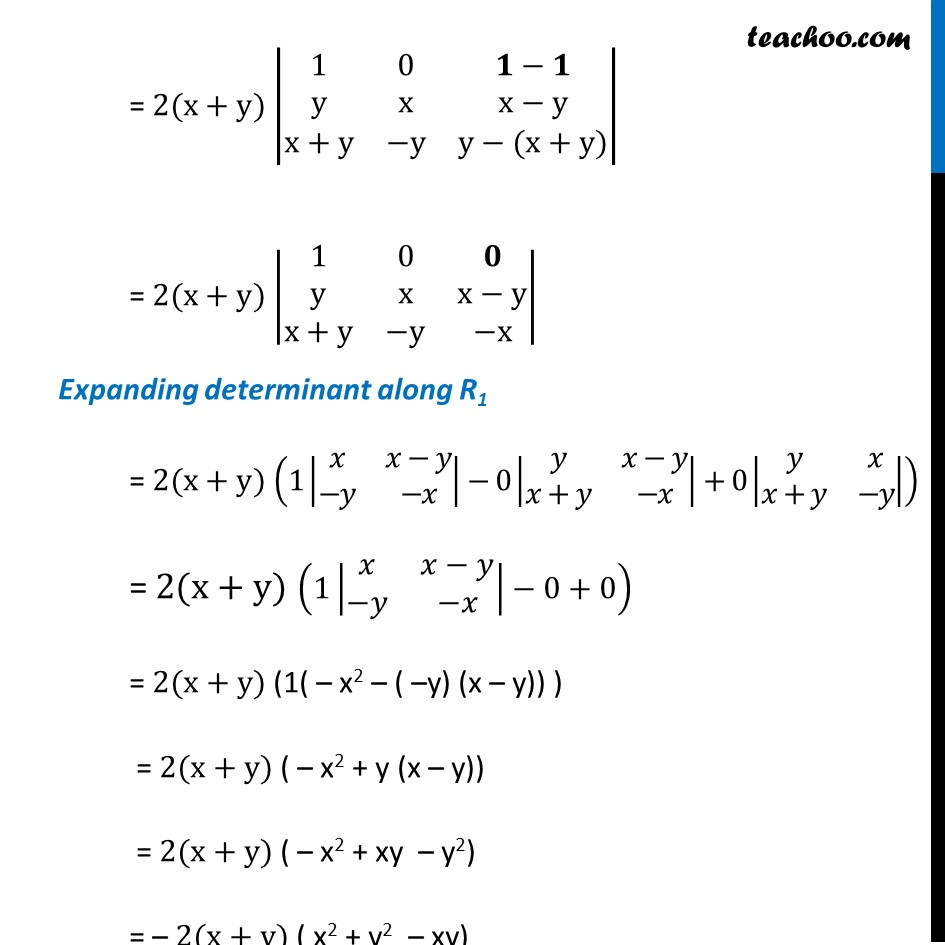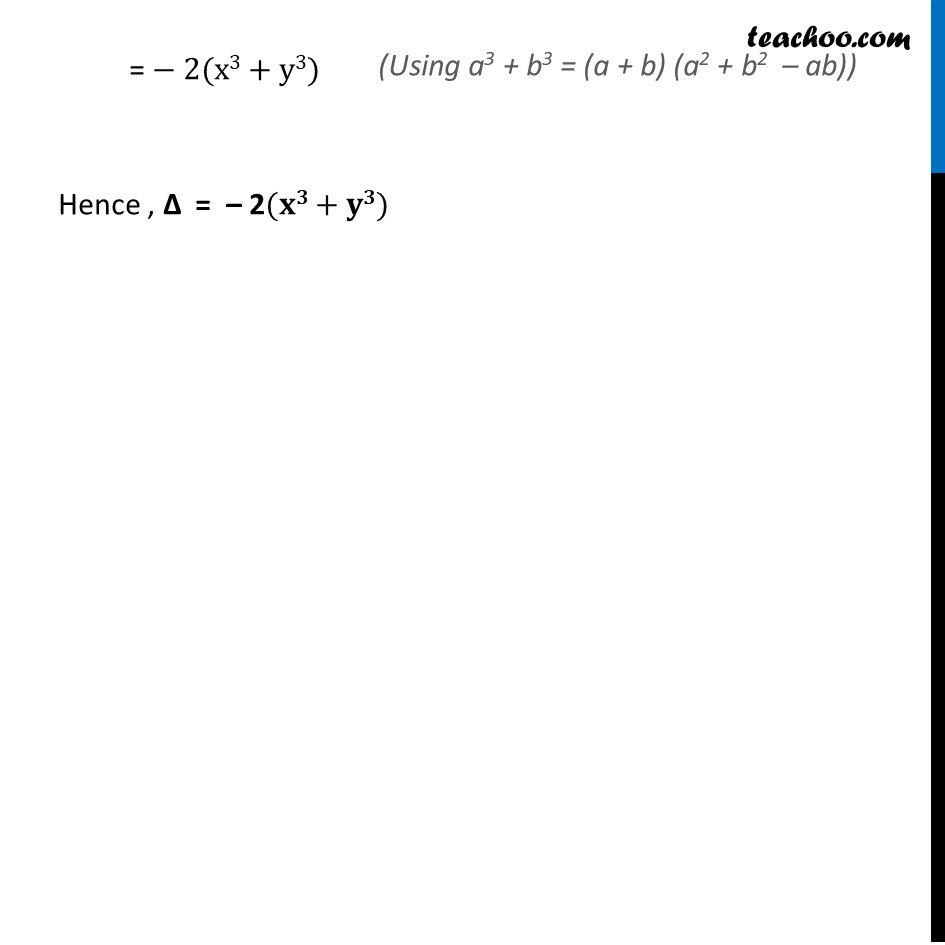Learn in your speed, with individual attention - Teachoo Maths 1-on-1 Class

### Transcript

Misc 5 (Method 1) Evaluate |■8(𝑥&𝑦&𝑥+𝑦@𝑦&𝑥+𝑦&𝑥@𝑥+𝑦&𝑥&𝑦)| Let ∆ = |■8(𝑥&𝑦&𝑥+𝑦@𝑦&𝑥+𝑦&𝑥@𝑥+𝑦&𝑥&𝑦)| = 𝑥[(𝑥+𝑦)𝑦−𝑥^2 ]−𝑦[𝑦^2−𝑥(𝑥+𝑦)]+(𝑥+𝑦)[𝑥𝑦−(𝑥+𝑦)^2 ] = 𝑥[𝑥𝑦+𝑦^2−𝑥^2 ]−𝑦[𝑦^2−𝑥^2−𝑥𝑦]+(𝑥+𝑦)[𝑥𝑦−𝑥^2−𝑦^2−2𝑥𝑦] = 𝒙[𝒙𝒚+𝒚^𝟐−𝒙^𝟐 ]−𝒚[𝒚^𝟐−𝒙^𝟐−𝒙𝒚]+(𝒙+𝒚)[−𝒙^𝟐−𝒚^𝟐−𝒙𝒚] = 𝑥^2 𝑦+𝑥𝑦^2−𝑥^3−𝑦^3+𝑥^2 𝑦+𝑥𝑦^2−𝑥^3−𝑥𝑦^2−𝑥^2 𝑦−𝑥^2 𝑦−𝑦[𝑦^2−𝑥^2−𝑥𝑦]+(𝑥+𝑦)[−𝑥^2−𝑦^2−𝑥𝑦] = 𝒙[𝒙𝒚+𝒚^𝟐−𝒙^𝟐 ]−𝒚[𝒚^𝟐−𝒙^𝟐−𝒙𝒚]+(𝒙+𝒚)[−𝒙^𝟐−𝒚^𝟐−𝒙𝒚] = 𝑥^2 𝑦+𝑥𝑦^2−𝑥^3−𝑦^3+𝑥^2 𝑦+𝑥𝑦^2−𝑥^3−𝑥𝑦^2−𝑥^2 𝑦−𝑥^2 𝑦−𝑦^3−𝑥𝑦^2 = 𝑥^2 𝑦+𝑥𝑦^2−𝑥^3−𝑦^3+𝑥^2 𝑦+𝑥𝑦^2−𝑥^3−𝑥𝑦^2−𝑥^2 𝑦−𝑥^2 𝑦−𝑦^3−𝑥𝑦^2 = −2𝑥^3−2𝑦^3 = − 2(x3+y3) Hence , ∆ = – 2(𝐱𝟑+𝐲𝟑) Misc 5 (Method 2) Evaluate |■8(𝑥&𝑦&𝑥+𝑦@𝑦&𝑥+𝑦&𝑥@𝑥+𝑦&𝑥&𝑦)| Let ∆ = |■8(𝑥&𝑦&𝑥+𝑦@𝑦&𝑥+𝑦&𝑥@𝑥+𝑦&𝑥&𝑦)| Applying R1→ R1 + R2 + R3 = |■8(𝑥+𝑦+𝑥+𝑦&𝑦+𝑥+𝑦+𝑥&𝑥+𝑦+𝑥+𝑦@𝑦&𝑥+𝑦&𝑥@𝑥+𝑦&𝑥&𝑦)| = |■8(2x+2y&2x+2y&2x+2y@y&x+y&x@x+y&x&y)| = |■8(𝟐(𝐱+𝐲)&𝟐(𝐱+𝐲)&𝟐(𝐱+𝐲)@y&x+y&x@x+y&x&y)| Taking common 2(x + y), from R1 = 𝟐(𝐱+𝐲) |■8(1&1&1@y&x+y&x@x+y&x&y)| Applying C2→ C2 – C1 = 2(x+y) |■8(1&𝟏−𝟏&1@y&x+y−𝑦&x@x+y&x−x−y&y)| = 2(x+y) |■8(1&𝟎&1@y&x&x@x+y&−y&y)| Applying C3 →C3 – C1 = 2(x+y) |■8(1&0&𝟏−𝟏@y&x&x−y@x+y&−y&y−(x+y))| = 2(x+y) |■8(1&0&𝟎@y&x&x−y@x+y&−y&−x)| Expanding determinant along R1 = 2(x+y) (1|■8(𝑥&𝑥−𝑦@−𝑦&−𝑥)|−0|■8(𝑦&𝑥−𝑦@𝑥+𝑦&−𝑥)|+0|■8(𝑦&𝑥@𝑥+𝑦&−𝑦)|) = 2(x+y) (1|■8(𝑥&𝑥−𝑦@−𝑦&−𝑥)|−0+0) = 2(x+y) (1( – x2 – ( –y) (x – y)) ) = 2(x+y) ( – x2 + y (x – y)) = 2(x+y) ( – x2 + xy – y2) = – 2(x+y) ( x2 + y2 – xy) = − 2(x3+y3) Hence , ∆ = – 2(𝐱𝟑+𝐲𝟑)